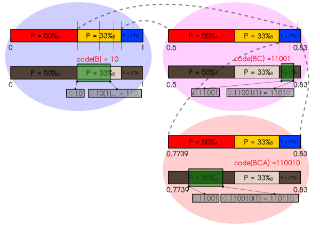# Introduction to Arithmetic Coding - Theory and Practice

Resource | v1 | created by janarez |
Type Paper
Created 2004-04-21
Identifier unavailable

Description

This introduction to arithmetic coding is divided in two parts. The first explains how and why arithmetic coding works. We start presenting it in very general terms, so that its simplicity is not lost under layers of implementation details. Next, we show some of its basic properties, which are later used in the computational techniques required for a practical implementation. In the second part, we cover the practical implementation aspects, including arithmetic operations with low precision, the subdivision of coding and modeling, and the realization of adaptive encoders. We also analyze the arithmetic coding computational complexity, and techniques to reduce it. We start some sections by first introducing the notation and most of the mathematical definitions. The reader should not be intimidated if at first their motivation is not clear: these are always followed by examples and explanations.

##### Relations

Arithmetic coding is a form of entropy encoding used in lossless data compression. Normally, a string...0.0 /10
useless alright awesome
##### from 0 reviews
Tip: Rating is anonymous unless you also write a comment.
Resource level 0.0 /10